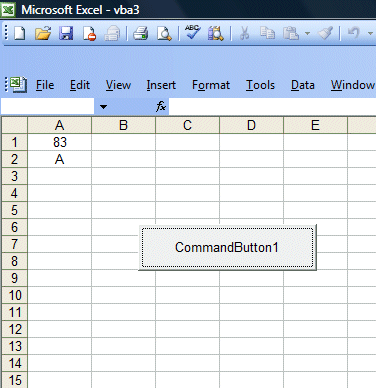Lesson 12: Using If...Then...Else

In this lesson, we shall learn how to create Excel VBA using If..Then...ElseIf statement to control program flow and enables decision making based on certain conditions. To control program flow, we use the If...Then...ElseIf structure together with conditional and logical operators.

12.1 Conditional and Logical Operators

To control the Visual Basic program flow, we can use various conditional operators. Basically, they resemble mathematical operators. Conditional operators are very powerful tools, they let the VB program compare data values and then decide what action to take, whether to execute a program or terminate the program and more. These operators are shown in Table 12.1.

Table 12.1: Conditional Operators
Meaning

=

Equal to

>

More than

<

Less Than

>=

More than or equal

<=

Less than or equal

<>

Not Equal to

Table 12.2:Logical Operators

Meaning

And

Both sides must be true

or

One side or other must be true

Xor

One side or other must be true but not both

Not

Negates truth

12.2 Using If.....Then.....Else   Statements  with Operators

To control the Visual Basic program flow, we shall use If...Then...Else statement together with the conditional operators and logical operators.
The syntax of the if...then...else statement is

If  conditions Then

VB expressions

ElseIf

VB expressions

ElseIf

VB expressions

Else

VB expressions

End If

* any If..Then..Else statement must end with End If. Sometime it is not necessary to use Else.

Example 12.1

In this example, you place the command button1 on the MS Excel spreadsheet and go into the VB editor by clicking on the button. At the Excel VB editor, key in the program codes as shown on the left.

I use the RND function to generate random numbers. In order to generate random integers between 0 and 100, I combined the syntax Int(Rnd*100). For example, when Rnd=0.6543, then Rnd*100=65.43, and Int(65.43)=65. Using the statement cells(1,1).Value=mark will place the value of 65 into cell(1,1).

Now, based on the mark in cells(1,1), I use the If...Then...Elseif statements to put the corresponding grade in cells(2,1). So, when you click on command button 1, it will put a random number between 1 and 100 in cells(1,1) and the corresponding grade in cells(2,1).

The InterfaceThe Code

```Private Sub CommandButton1_Click()

Dim mark As Integer
mark = Int(Rnd * 100)
Cells(1, 1).Value = mark
If mark < 20 And mark >= 0 Then
ElseIf mark < 30 And mark >= 20 Then
ElseIf mark < 40 And mark >= 30 Then
ElseIf mark < 50 And mark >= 40 Then
ElseIf mark < 60 And mark >= 50 Then
ElseIf mark < 70 And mark >= 60 Then
ElseIf mark < 80 And mark >= 70 Then